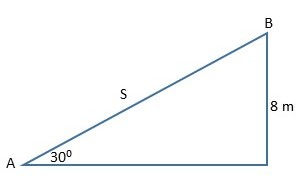# An object of mass 4.0 kg starts at rest from the top of a rough inclined plane of height 8.0 m as...

## Question:

An object of mass 4.0 kg starts at rest from the top of a rough inclined plane of height 8.0 m as shown in the figure. If the speed of the object at the bottom of the inclined plane is 10 m/s, how much work does the friction do on this object as it slides down the incline?## Conservation of Energy:

The kinetic energy of a body with mass m and speed v is

{eq}K = \frac{1}{2} m v^2. {/eq}

The gravitational potential energy of the body when raised by a height h is

{eq}U = mgh, {/eq}

where g is the acceleration due to gravity.

The total mechanical energy of the body is the sum of its kinetic and potential energies. Conservation of energy requires that the final mechanical energy is equal to the initial mechanical energy plus work done on the body, W:

{eq}K_f + U_f = K_i + U_i + W, {/eq}

where the f and i subscripts indicate the final and initial values, respectively.

## Answer and Explanation:

As the object starts from rest, its initial kinetic energy is 0. At the bottom of the incline, the final kinetic energy is

{eq}K_f = \frac{1}{2} m v^2, {/eq}

where m = 4.0 kg is its mass, and v = 10 m/s is its speed.

If we set the gravitational potential energy to a reference value of 0 at the bottom of the incline, the object's initial potential energy is

{eq}U_i = mgh, {/eq}

where h = 8.0 m is its height, and {eq}g = 9.8\,\mathrm{m/s^2} {/eq} is the acceleration due to gravity.

Conservation of energy then gives:

{eq}U_i + W = K_f, {/eq}

where W is the work done by friction on the body.

{eq}mgh + W = \frac{1}{2} mv^2 \\ W = m(\frac{1}{2} v^2 - gh) \\ W = 4.0\,\text{kg} \times ( \frac{1}{2} (10\,\text{m/s})^2 - 9.8\,\mathrm{m/s^2} \times 8.0\,\text m) \\ W = -114\,\text J {/eq}

The work done by friction on the object is -114 J.